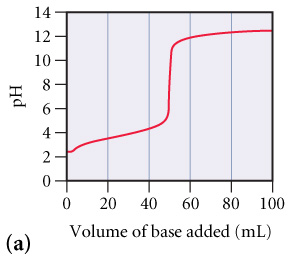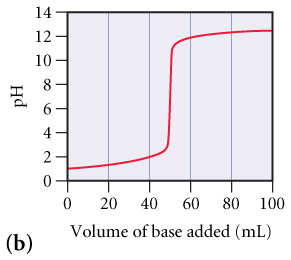# Problem: The graphs below labeled (a) and (b) show the titration curves for two equal-volume samples of monoprotic acids, one weak and one strong. Both titrations were carried out with the same concentration of strong base.Part AWhat is the approximate pH at the equivalence point of (a) curve? (Figure 1)Express your answer as a whole number?Part BWhat is the approximate pH at the equivalence point of (b) curve?(Figure 2)Express your answer as a whole number.Part CWhich curve corresponds to the titration of the strong acid and which one to the titration of the weak acid?A. Graph (a) represents a weak acid and graph (b) represents a strong acid.B. Graph (b) represents a weak acid and graph (a) represents a strong acid.

###### FREE Expert Solution

Recall that

• Weak acid and strong base titration
• pH gradually increases as it approaches the equivalence point
• pH at the equivalence point is more than 7.00
• Strong acid and strong base titration
• pH rapidly increases as it approaches the equivalence point
• pH at the equivalence point is equal to 7.00

Part A.

We will approximate the pH by determining the pH that corresponds to the volume at the equivalence point

82% (450 ratings)###### Problem Details

The graphs below labeled (a) and (b) show the titration curves for two equal-volume samples of monoprotic acids, one weak and one strong. Both titrations were carried out with the same concentration of strong base.

Part A

What is the approximate pH at the equivalence point of (a) curve? (Figure 1)Part B

What is the approximate pH at the equivalence point of (b) curve?(Figure 2)Part C

Which curve corresponds to the titration of the strong acid and which one to the titration of the weak acid?

A. Graph (a) represents a weak acid and graph (b) represents a strong acid.

B. Graph (b) represents a weak acid and graph (a) represents a strong acid.